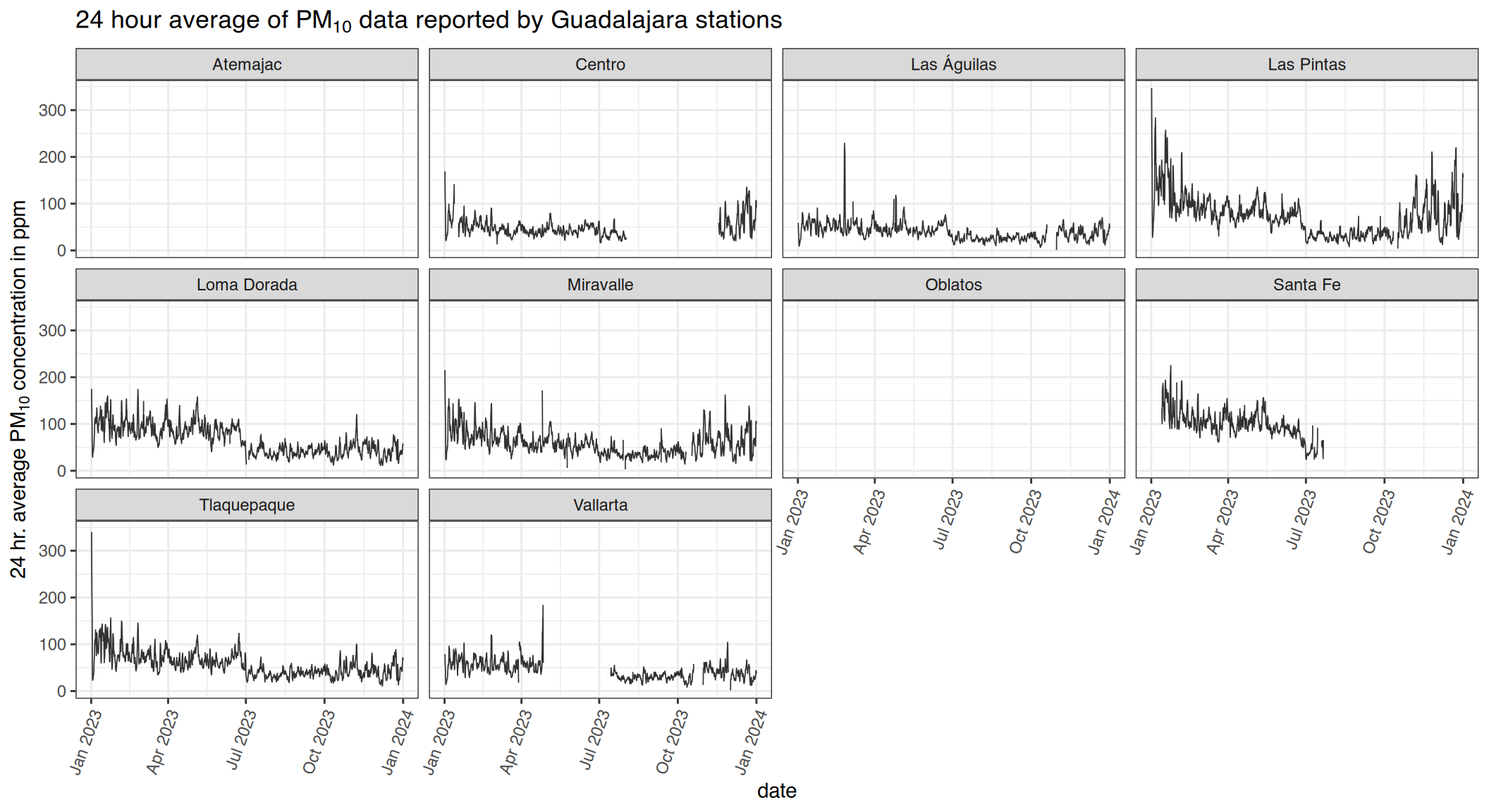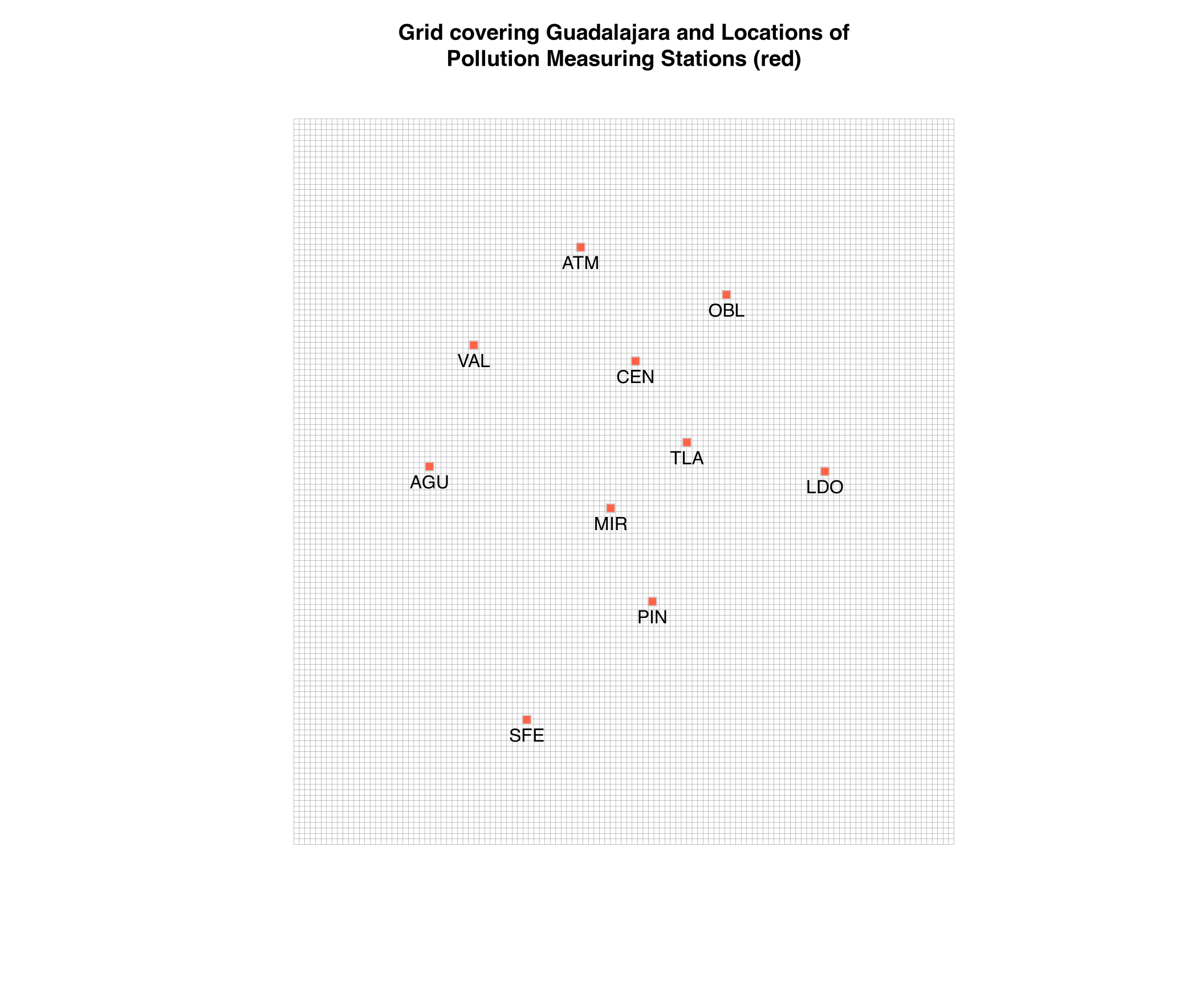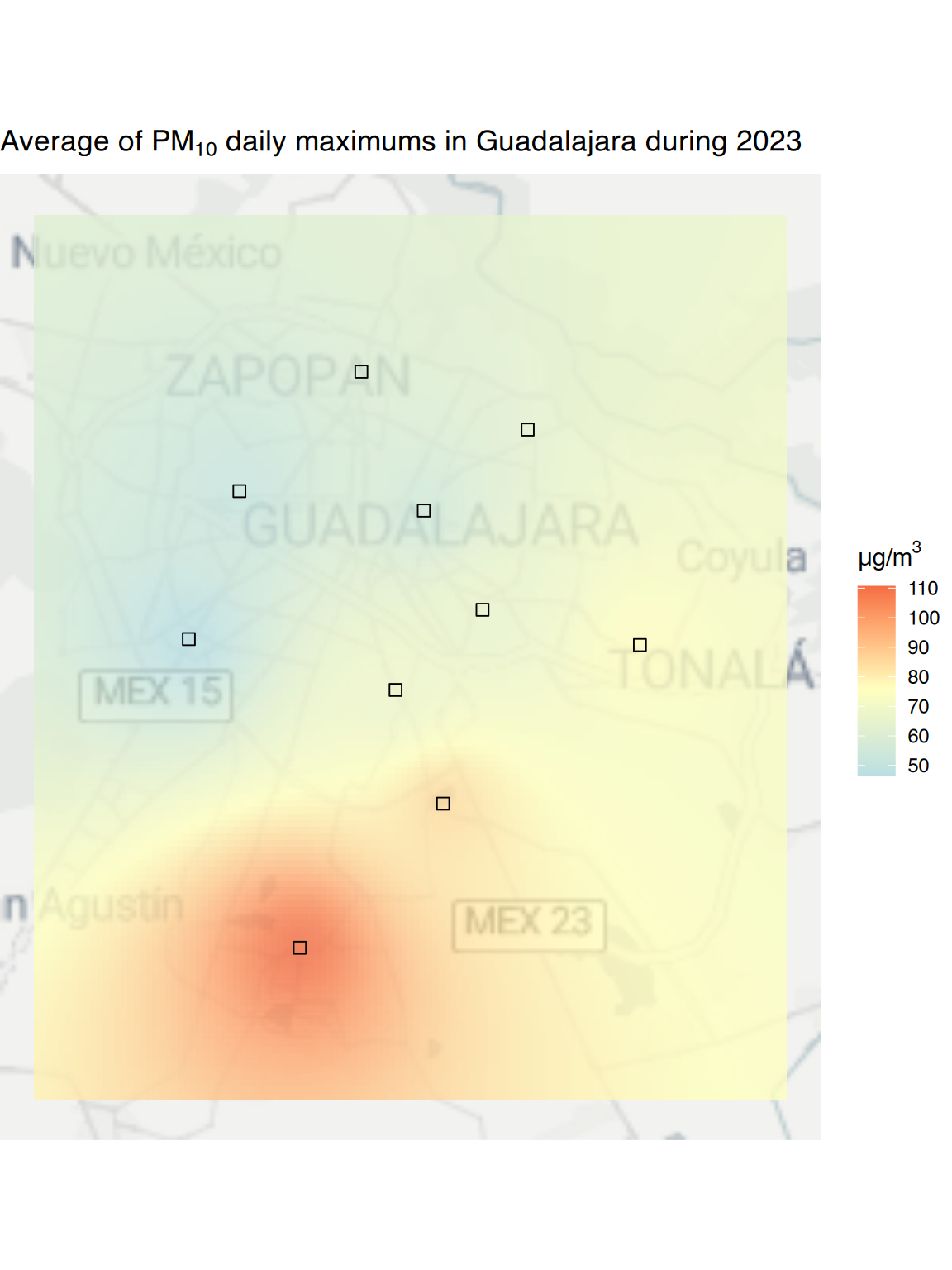The Instituto Nacional de Ecología y Cambio Climático (INECC) created the SINAICA website to gather the information generated by all the air quality monitoring stations located throughout Mexico. The pollution and meteorological information is generated by the monitoring stations run by the local authorities, who then trasmit it to SINAICA. This R package provides tools for downloading that information, and in this example we'll use it to create a simple map of PM10 pollution in Guadalajara.

First, load the packages necessary for running the analysis

## Auto-install required R packages
packs <- c("dplyr", "ggplot2", "lubridate", "sp", "ggmap", "gstat", "zoo",
"tidyr", "rsinaica")
success <- suppressWarnings(sapply(packs, require, character.only = TRUE))
if (length(names(success)[!success])) {
install.packages(names(success)[!success])
sapply(names(success)[!success], require, character.only = TRUE)
}

## Core Functions

The basic set of functions provided by the package:

• sinaica_station_data: Download data from a single station by specifying a parameter and a date range

• sinaica_param_data: Download data from all stations for a single parameter by specifying a date range

Both functions can download the crude real-time data as it comes in, the older and not as complete validated data, or pollution data which is collected manually and sent to an external for lab analysis.

We'll use the sinaica_station_data function to download pollution data for all the stations located in Guadalajara. We'll also use the crude real-time data since there is no validated data in Guadalajara for 2017, and ozone samples are not collected manually in any of the stations.

## Change this if you want a map of another city or parameter
parameter <- "PM10"
midpoint <- 76 # midpoint of regular air quality converted to IMECAs

get_month <- function(start_date, end_date, net, parameter){
bind_rows(
lapply(stations_sinaica$station_id[stations_sinaica$network_name %in% net],
sinaica_station_data, parameter, start_date, end_date, "Crude")
)
}
df <- bind_rows(
mapply(get_month,
seq(as.Date("2017-01-01"), as.Date("2017-12-01"), by = "month"),
seq(as.Date("2017-02-01"), as.Date("2018-01-01"), by = "month") - 1,
network,
parameter,
SIMPLIFY = FALSE)
)

knitr::kable(head(df))
station_id station_name date hour valid unit value
103 Las Águilas 2017-01-01 1 1 µg/m³ 19.053
103 Las Águilas 2017-01-01 2 1 µg/m³ 35.795
103 Las Águilas 2017-01-01 3 1 µg/m³ 45.147
103 Las Águilas 2017-01-01 4 1 µg/m³ 34.222
103 Las Águilas 2017-01-01 5 1 µg/m³ 41.951
103 Las Águilas 2017-01-01 6 1 µg/m³ 33.367

Usually, for PM10 particles, a 24-hour average is taken as the current pollution value.

df <- df  %>%
ungroup() %>%
complete(station_id,
hour = 0:23,
date = as.character(seq(as.Date("2017-01-01"), as.Date("2017-12-31"), by = "day"))) %>%
arrange(station_id, date, hour) %>%
ungroup() %>%
group_by(station_id) %>%
mutate(roll24 = rollapply(value, 24, mean, na.rm = TRUE, partial = 12,
fill = NA, align = "right")) %>%
select(-station_name) %>%
left_join(stations_sinaica[,c("station_id", "station_name")], by = "station_id") %>%
mutate(datetime = with_tz(as.POSIXct(paste0(date, " ", hour, ":00"),
tz = "Etc/GMT+6"),
tz = "America/Mexico_City"))

ggplot(df, aes(datetime, roll24, group = station_name)) +
geom_line(alpha = .8, size = .3) +
ggtitle(expression(paste("24 hour average of ", PM, " data reported by Guadalajara stations"))) +
xlab("date") +
ylab(expression(paste("24 hr. average ", PM," concentration in ppm"))) +
facet_wrap(~ station_name) +
theme_bw() +
theme(axis.text.x=element_text(angle=70,hjust=1))
#> Warning: Removed 3100 row(s) containing missing values (geom_path).We will use the longitude and latitude data of the measuring stations in the stations_sinaica data.frame to create a grid covering Guadalajara, and then extrapolate the pollution values to each cell in the grid by inverse distance weighting from the values in the locations of the measuring stations.

create_grid <- function(station_vec, pixels = 1000) {
df <- stations_sinaica[stations_sinaica$station_id %in% station_vec,] geog <- df[,c("lat", "lon")] coordinates(geog) <- ~lon+lat proj4string(geog) <- "+proj=longlat +datum=WGS84 +no_defs +ellps=WGS84 +towgs84=0,0,0" ## Add a margin surrounding the stations geog@bbox <- bbox(geog) + c(-0.05, -0.05, 0.05, 0.05) geog.grd <- makegrid(geog, n = pixels) grd.pts <- SpatialPixels(SpatialPoints(geog.grd)) as(grd.pts, "SpatialGrid") } reporting_stations <- unique(df$station_id)
# 50x50 grid covering Mexico City
grid <- create_grid(reporting_stations, 15000)
#> Warning in showSRID(uprojargs, format = "PROJ", multiline = "NO"): Discarded datum WGS_1984 in CRS definition,
#>  but +towgs84= values preserved

plot(grid, main = "Grid covering Guadalajara and Locations of\nPollution Measuring Stations (red)",
col = "#666666", lwd = .2)
# Plot the locations of the stations
geog <- stations_sinaica[stations_sinaica$station_id %in% reporting_stations, c("lat", "lon", "station_code")] coordinates(geog) <- ~lon+lat points(geog, pch = 22, col = "lightgray", bg = "tomato1", cex = 1.2) text(geog$lon, geog$lat, geog$station_code, pos = 1)Now that the grid is ready we can use inverse distance to calculate the pollution values in each grid cell.

heatmap <- function(df, grid){
if(nrow(df) == 0){
return(data.frame(var1.pred = NA, var1.var = NA, lon = NA, lat = NA))
}

df <- left_join(df, stations_sinaica, by = "station_id")
df <- df[!is.na(df$value),] df <- df[,c("lat", "lon", "value")] coordinates(df) <- ~lon+lat # For radiation pollution the exponent should be 2 # See http://www.sciencedirect.com/science/article/pii/S009830041200372X df.idw <- idw(value ~ 1, df, grid, idp = 2, debug.level = 0) idw = as.data.frame(df.idw) names(idw) <- c("var1.pred", "var1.var", "lon", "lat") idw } We'll show on the map the average of all the daily maximums of the twenty-four-hour rolling average of PM10 values. df <- df %>% group_by(station_id, date) %>% summarise(maxroll24 = max(roll24, na.rm = TRUE)) %>% mutate(maxroll24 = if_else(!is.finite(maxroll24), NA_real_, maxroll24)) %>% group_by(station_id) %>% summarise(value = mean(maxroll24, na.rm = TRUE)) #> summarise() regrouping output by 'station_id' (override with .groups argument) #> summarise() ungrouping output (override with .groups argument) idw_tiles <- heatmap(df, grid) ## Plot Now we will use the package ggmap to plot the locations of the stations and the pollution grid on top of a map. qmplot(x1, x2, data = data.frame(grid), geom = "blank", maptype = "toner-lite", source = "stamen", zoom = 10) + geom_tile(data = idw_tiles, aes(x = lon, y = lat, fill = var1.pred), alpha = .8) + ## set midpoint at 76 cause that's bad air quality according to ## http://www.aire.cdmx.gob.mx/default.php?opc=%27ZaBhnmI=&dc=%27aQ== scale_fill_gradient2(expression(paste(mu,"g/", m^3)), low = "#abd9e9", mid = "#ffffbf", high = "#f46d43", midpoint = midpoint) + # midpoint of regular air quality converted from IMECAs geom_point(data = stations_sinaica[stations_sinaica$station_id %in% reporting_stations, ],
aes(lon, lat), shape = 22, size = 2.6) +
ggtitle(expression(paste("Average of ", PM,
" daily maximums in Guadalajara during 2017")))